NCERT Solutions: Quadratic Equations (Exercise 4.1)

# NCERT Solutions: Quadratic Equations (Exercise 4.1) - Notes | Study Mathematics (Maths) Class 10 - Class 10

 1 Crore+ students have signed up on EduRev. Have you?

Q1. Check whether the following are quadratic equations:

(i) (x + 1)2 = 2(x − 3)
(ii) x2 − 2x = (− 2) (3 − x)
(iii) (x − 2) (x + 1) = (x − 1) (x + 3)
(iv) (x − 3) (2x + 1) = x(x + 5)
(v) (2x − 1) (x − 3) = (x + 5) (x − 1)
(vi) x2 + 3x + 1 = (x − 2)2
(vii) (x + 2)3 = 2x(x2 − 1)
(viii) x3 − 4x2 − x + 1 = (x − 2)
3

Ans: (i) (x + 1)2 = 2(x−3)

We have:

(x + 1)2 = 2 (x − 3)
⇒ x+ 2x + 1 = 2x − 6
⇒ x2 + 2x + 1 − 2x + 6 = 0
⇒ x2 + 7 = 0

Since x2 + 7 is a quadratic polynomial
∴ (x + 1)2 = 2(x − 3) is a quadratic equation.

(ii) x2−2x = (− 2) (3 −x)

We have:

x2 − 2x = (− 2) (3 − x)
⇒ x2 − 2x = − 6 + 2x
⇒ x2 − 2x − 2x + 6 = 0
⇒ x2 − 4x + 6 = 0

Since x− 4x + 6 is a quadratic polynomial
∴ x2 − 2x = (−2) (3 − x) is a quadratic equation.

(iii) (x−2) (x + 1) = (x−1) (x + 3)

We have:

(x − 2) (x + 1) = (x − 1) (x + 3)
⇒ x2 − x − 2 = x2 + 2x − 3
⇒ x2 − x − 2 − x2 − 2x + 3 = 0
⇒ −3x + 1 = 0

Since −3x + 1 is a linear polynomial

∴ (x − 2) (x + 1) = (x − 1) (x + 3) is not quadratic equation.

(iv) (x−3) (2x + 1) = x(x + 5)

We have:

(x − 3) (2x + 1) = x (x + 5)
⇒ 2x2 + x − 6x −3= x+ 5x
⇒ 2x2 − 5x − 3 − x2 − 5x =0
⇒ x2 + 10x − 3= 0

Since x2 + 10x − 3 is a quadratic polynomial

∴ (x − 3) (2x + 1) = x(x + 5) is a quadratic equation.

(v) (2x−1) (x−3) = (x + 5) (x−1)

We have:

(2x − 1) (x − 3) = (x + 5) (x − 1)

⇒ 2x2 − 6x − x + 3 = x2 − x + 5x − 5

⇒ 2x2 − x2 − 6x − x + x − 5x + 3 + 5 = 0
⇒ x2 − 11x + 8 = 0

Since x2 − 11x + 8 is a quadratic polynomial

∴ (2x − 1) (x − 3) = (x + 5) (x − 1) is a quadratic equation.

(vi) x2 + 3x + 1 = (x−2)2

We have:

x2 + 3x + 1 = (x − 2)2
⇒ x2 + 3x + 1 = x2 − 4x + 4
⇒ x2 + 3x + 1 − x+ 4x −4= 0
⇒ 7x − 3 = 0

Since 7x − 3 is a linear polynomial

∴ x2 + 3x + 1 = (x − 2)is not a quadratic equation.

(vii) (x + 2)3 = 2x(x2−1)

We have:

(x + 2)3 = 2x(x2 − 1)
⇒ x3 + 3x2(2) + 3x(2)2 + (2)3 = 2x3 − 2x
⇒ x3 + 6x2 + 12x + 8 = 2x3 − 2x
⇒ x3 + 6x2 + 12x + 8 − 2x3 + 2x = 0
⇒− x3 + 6x2 + 14x + 8 = 0
Since −x3 + 6x2 + 14x + 8 is a polynomial of degree 3
∴ (x + 2)3 = 2x(x2 − 1) is not a quadratic equation.

(viii) x3−4x2−x + 1 = (x− 2)3

We have:

x3 − 4x2 − x + 1 = (x − 2)3
⇒ x3 − 4x2 − x + 1 = x3 + 3x2(− 2) + 3x(− 2)2 + (− 2)3
⇒ x3 − 4x2 − x + 1 = x3 − 6x2 + 12x − 8
⇒ x3 − 4x2 − x − 1 − x3 + 6x2 − 12x + 8 = 0
⇒ 2x2 − 13x + 9 = 0

Since 2x2 − 13x + 9 is a quadratic polynomial

∴ x3 − 4x2 − x + 1 = (x − 2)3 is a quadratic equation.

Q2. Represent the following situations in the form of quadratic equations:
(i) The area of a rectangular plot is 528 m2. The length of the plot (in metres) is one more than twice its breadth. We need to find the length and breadth of the plot.
(ii) The product of two consecutive positive integers is 306. We need to find the integers.
(iii) Rohan’s mother is 26 years older than him. The product of their ages (in years) 3 years from now will be 360. We would like to find Rohan’s present age.
(iv) A train travels a distance of 480 km at a uniform speed. If the speed had been 8 km/h less, then it would have taken 3 hours more to cover the same distance. We need to find the speed of the train.

Ans: (i) Let the breadth = x metres
∵ Length = 2 (Breadth) + 1

∴ Length = (2x + 1) metres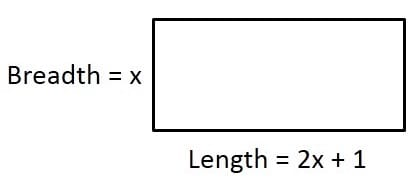Since Length × Breadth = Area

∴ (2x + 1) × x = 528
⇒ 2x2 + x = 528
⇒ 2x2 + x − 528 = 0
Thus, the required quadratic equation is 2x2 + x− 528 = 0

(ii) Let the two consecutive numbers be x and (x + 1).
∵Product of the numbers = 306
∴ x (x + 1) = 306
⇒ x2 + x = 306
⇒ x2 + x − 306 = 0

Thus, the required equdratic equation is x2 + x − 306 = 0

(iii) Let the present age = x
∴ Mother’s age = (x + 26) years

After 3 years

His age = (x + 3) years
Mother’s age = [(x + 26) + 3] years
=(x + 29) years

According to the condition,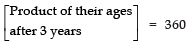⇒ (x + 3) × (x + 29) = 360
⇒ x2 + 29x + 3x + 87 = 360
⇒ x2 + 29x + 3x + 87 − 360 = 0
⇒ x2 + 32x − 273 = 0

Thus, the required quadratic equation is

x2 + 32x − 273 = 0

(iv) In first case,

Let the speed of the train = u km/hr
Distance covered = 480 km
Time taken = Distance ÷ Speed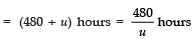In second case,

Speed = (u − 8) km/hour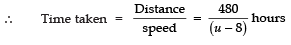According to the condition,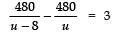⇒ 480u − 480(u − 8) = 3u(u − 8)
⇒ 480u − 480u + 3840 = 3u2 − 24u
⇒ 3840 − 3u2 + 24u =0
⇒ 1280 − u+ 8u =0
⇒ − 1280 + u2 − 8u =0
⇒ u2 − 8u − 1280 = 0

Thus, the required quadratic equation is u2 − 8u – 1280 = 0

The document NCERT Solutions: Quadratic Equations (Exercise 4.1) - Notes | Study Mathematics (Maths) Class 10 - Class 10 is a part of the Class 10 Course Mathematics (Maths) Class 10.
All you need of Class 10 at this link: Class 10

## Mathematics (Maths) Class 10

53 videos|403 docs|138 tests
 Use Code STAYHOME200 and get INR 200 additional OFF

## Mathematics (Maths) Class 10

53 videos|403 docs|138 tests

Track your progress, build streaks, highlight & save important lessons and more!

,

,

,

,

,

,

,

,

,

,

,

,

,

,

,

,

,

,

,

,

,

;